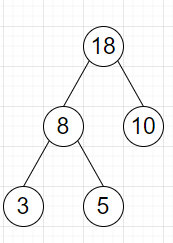# Program to check whether each node value except leaves is sum of its children value or not in Python

Suppose we have a binary tree, we have to check whether for every node in the tree except leaves, its value is same as the sum of its left child's value and its right child's value or not.

So, if the input is likethen the output will be True

To solve this, we will follow these steps −

• Define a function dfs() . This will take root

• if root is null, then

• return True

• if left of root is null and right of root is null, then

• return True

• left := 0

• if left of root is not null, then

• left := value of left of root

• right := 0

• if right of root is not null, then

• right := value of right of root

• return true when (left + right is same as value of root) and dfs(left of root) is true and dfs(right of root) is true

• From the main method do the following −

• return dfs(root)

Let us see the following implementation to get better understanding −

## Example

Live Demo

class TreeNode:
def __init__(self, data, left = None, right = None):
self.val = data
self.left = left
self.right = right
class Solution:
def solve(self, root):
def dfs(root):
if root == None:
return True
if root.left == None and root.right == None:
return True
left = 0
if root.left:
left = root.left.val
right = 0
if root.right:
right = root.right.val
return (left + right == root.val) and dfs(root.left) and
dfs(root.right)
return dfs(root)
ob = Solution()
root = TreeNode(18)
root.left = TreeNode(8)
root.right = TreeNode(10)
root.left.left = TreeNode(3)
root.left.right = TreeNode(5)
print(ob.solve(root))

## Input

root = TreeNode(18) root.left = TreeNode(8) root.right = TreeNode(10)
root.left.left = TreeNode(3) root.left.right = TreeNode(5)

## Output

True